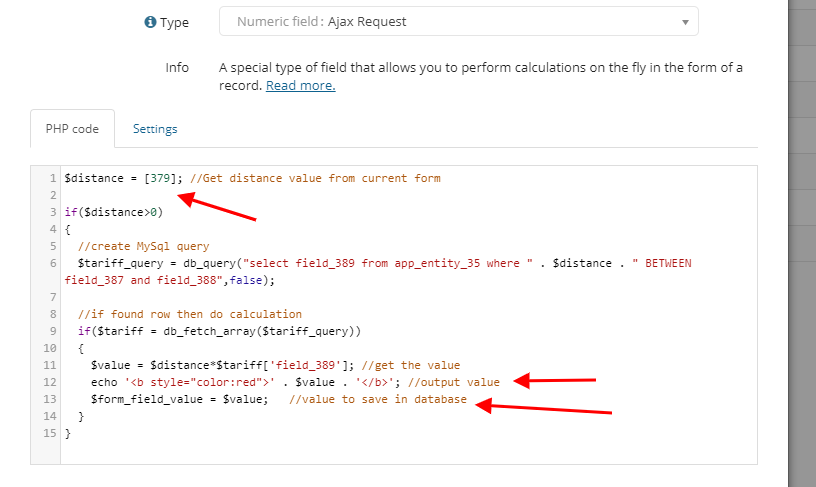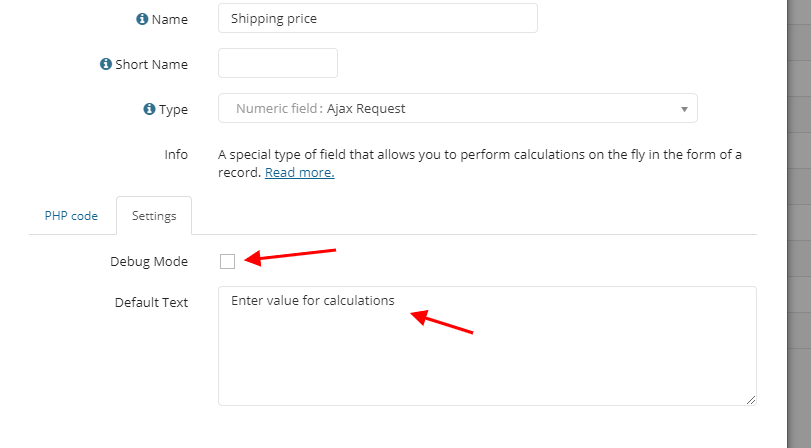A special type of field that allows you to perform calculations on the fly in a record form. In the "PHP code" option, enter your own code that will be executed when opening the form. If the code uses values from the form, then when the value in the input field is changed, the code will be executed again.# Working with values

To get a value from the form, use the following construction: `[field id]`. For example: `\$distance = ;`
For a nested entity, you can get the value from the parent record in the same way.

Note: if the values of field 379 are empty, then `\$distance = 0;.`

To get the id of the current record, use `\$item_id = \$_GET['item_id'];`
`\$_POST['parent_item_id']` - id of the parent record

Use the "Debug mode" option to check the code that will be executed.If the default text is entered, it will be displayed when an ajax request returns an empty response.

# Output and save the value in the database

Use the `echo`  command to output the value. The output can be in any format, such as `echo '<b style= "color:red">' . \$value . '</b>';`

To save the value in the database, assign the value to a special variable `\$form_field_value`, for example `\$form_field_value = \$value;`

# JavaScript

If you need to assign the received value to another field in the form, you can do this using JavaScript code. Example:

`echo '<script>\$("#fields_13").val(' . \$value . ')</script>';`

Where 13 is the field id. \$value - the required value.

# MySql query

Using the `db_query()` function, you can build a query to the required table and select the values you need. For example:

`\$tariff_query = db_query("select field_389 from app_entity_35 where " . \$distance . " BETWEEN field_387 and field_388",false);`

# Full Example

```\$distance = ; //Get distance value from current form

if(\$distance>0)
{
//create MySql query
\$tariff_query = db_query("select field_389 from app_entity_35 where " . \$distance . " BETWEEN field_387 and field_388",false);

//if found row then do calculation
if(\$tariff = db_fetch_array(\$tariff_query))
{
\$value = \$distance*\$tariff['field_389']; //get the value
echo '<b style="color:red">' . \$value . '</b>'; //output value
\$form_field_value = \$value;   //value to save in database
}
}```

# Working with dates

If you need to select data for a specific date in the request, use the FROM_UNIXTIME(unix_timestamp,format) function to compare dates, for example:

`\$tariff_query = db_query("select field_389 from app_entity_35 where FROM_UNIXTIME(field_387,'%Y-%m-%d')=FROM_UNIXTIME( ,'%Y-%m-%d')",false);`

`field_387` - this is the date field in the entity where are you looking for
`400` - this is the date field in the form

# Drop-down list value

Example of a request to get a value from a drop-down list:

```\$value = '';
\$choices_id = ; //Get the ID of the selected item from the list
/*
Building a query to get a value from the list to the app_fields_choices table
Field 157-drop-down list
*/
\$item_query = db_query("select value from app_fields_choices where fields_id=157 and id={\$choices_id}");
if(\$item = db_fetch_array(\$item_query))
{
\$value = \$item['value'];
}

echo \$value; ```

# Show image

Example to output image from record by record ID:

```\$item_id = ; //get record id

//field_571 is Image Field in Entity 49
\$item_info_query = db_query("select field_571 from app_entity_49 where id='{\$item_id}'");
if(\$item_info = db_fetch_array(\$item_info_query))
{
\$img = \$item_info['field_571'];

\$file = attachments::parse_filename(\$img); //get attachment info

//check if file exist and output image
if(is_file(\$file['file_path']))
{
echo '<img src="data:image/jpeg;base64,' . base64_encode(file_get_contents(\$file['file_path'])) . '" width="200">';
}
}```